﻿﻿﻿

## Heat Transfer simulation tasks

### Heat transfer enhancement for heat exchanger elements

 Heat transfer enhancement for heat exchanger elements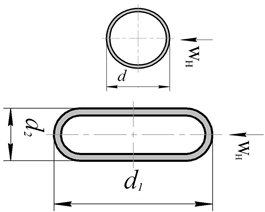Experiment at Re=15 000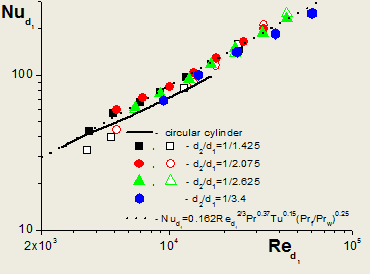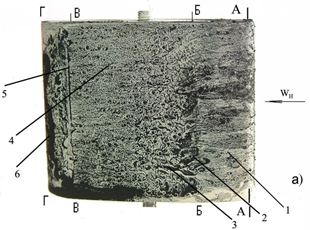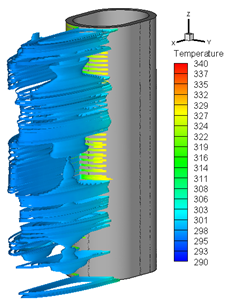Comparison of oval-shaped cylinders with circular ones Modeling

 Heat transfer enhancement for LED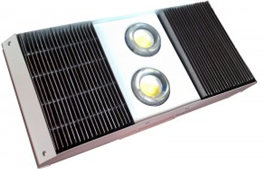Conjugated problem of heat removal from LED Heat removal system contains heat pipes Used software: Ansys Fluent 14.5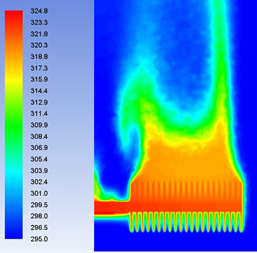Source Power = 90 Wt Ambient T = 295 K Heat pipe thermal cond. =25000 Wt/mK Temperature distribution at the heat removal system surface Heat wake above LED heat removal system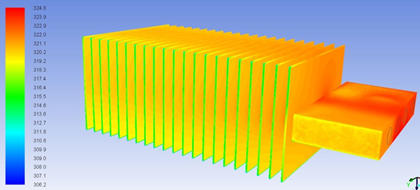Thermal regime of control system microelement of car generator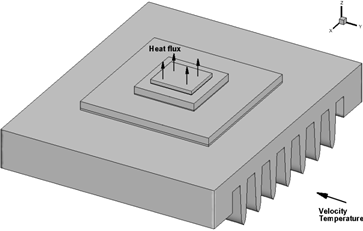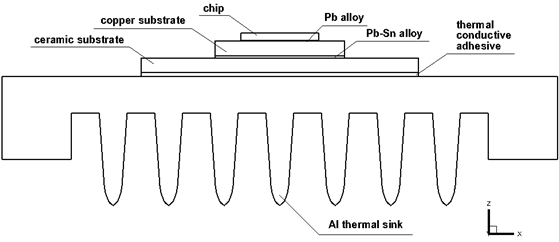Surface of: chip – 5x5 mm, copper substrate – 8x8 mm, ceramic substrate – 15x15 mm, Al thermal sink – 25x30 mm Boundary conditions: Heat power of the chip – 3 Wt, Inlet air velocity – 5 m/s, Inlet air temperature – 398 K, Inlet turbulence level – 10% Used software: Ansys Fluent 14.5

#### Thermal regime of control system microelement of car generator

Q.: Which of the variants gives a minimal chip temperature?

Nos Properties Averaged temperature of the chip surface Maximal temperature of the chip
1 Copper – 7x7 mm, copper λ=150 Wt/(m K), adhesive λ=0.88 Wt/(m K) 430.44 K (157.44 C) 430.93 К (157.93 C)
2 Copper – 7x7 mm, change in ceramics Al2O3 to Al of grade AD0 with ρ=2710 kg/m3, λ=202.4 Wt/(m K), сp=930 J/(kg K); copper λ=380 Wt/(m K), adhesive λ=1.68 Wt/(m K) 421.74 К (148.74 С) 421.97 К (148.97 С)
3 Copper – 8x8 mm, copper λ=380 Wt/(m K), adhesive λ=0.88 Wt/(m K) 423.59 K (150.59 C) 423.87 K (150.87 C)
4 Copper – 8x8 mm, copper λ=380 Wt/(m K), adhesive λ=1.68 Wt/(m K) 421.66 K (148.66 C) 421.93 K (148.93 C)

 Variant 1: Copper – 7x7 mm, copper λ=150 Wt/(m K), adhesive λ=0.88 Wt/(m K)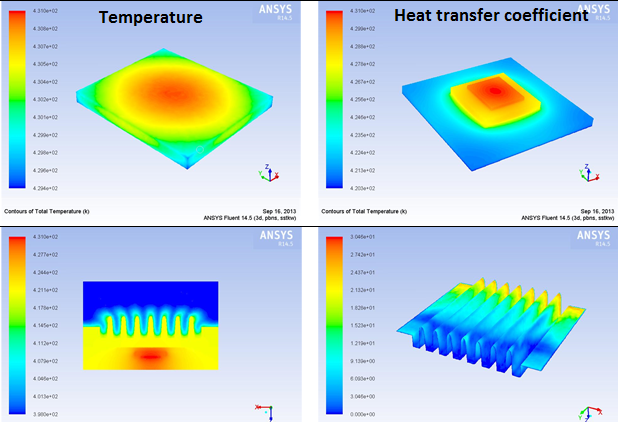Variant 2: Copper – 7x7 mm, change in ceramics Al2O3 to Al with grade AD0 (ρ=2710 kg/m3, λ=202.4 Wt/(m K), сp=930 J/(kg K)); copper λ=380 Wt/(m K), adhesive λ=1.68 Wt/(m K)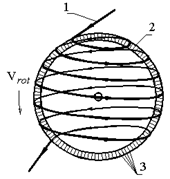3D problem using the CAD model close to a real device Unsteady RANS equations, SST–k–ω turbulence model, upwind scheme, SIMPLEС algorithm Used software: Ansys Fluent 15.0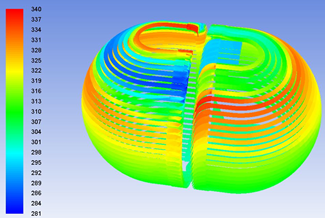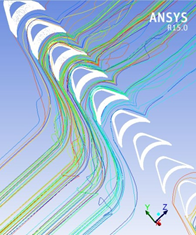CAD-model ‘Turbosphere’ Temperature distribution in the gas pipeline Streamlines near blades